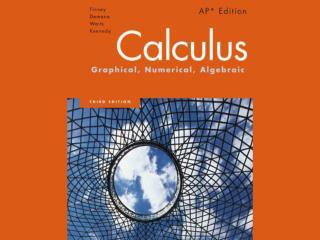# Chapter 1 - PowerPoint PPT PresentationDownload PresentationChapter 1

Chapter 1Download Presentation## Chapter 1

- - - - - - - - - - - - - - - - - - - - - - - - - - - E N D - - - - - - - - - - - - - - - - - - - - - - - - - - -
##### Presentation Transcript

1. Chapter 1 Prerequisites for Calculus

2. 1.1 Lines

3. Quick Review

4. Quick Review

5. Quick Review

6. Quick Review Solutions

7. Quick Review Solutions

8. Quick Review Solutions

9. What you’ll learn about… • Increments • Slope of a Line • Parallel and Perpendicular Lines • Equations of Lines • Applications …and why. Linear equations are used extensively in business and economic applications.

10. Increments

11. Example Increments

12. Slope of a Line A line that goes uphill as x increases has a positive slope. A line that goes downhill as x increases has a negative slope.

13. Slope of a Line

14. Parallel and PerpendicularLines

15. Equations of Lines

16. Example Equations of Lines

17. Point Slope Equation

18. Example: Point Slope Equation

19. Equations of Lines

20. Slope-Intercept Equation

21. General Linear Equation Although the general linear form helps in the quick identification of lines, the slope-intercept form is the one to enter into a calculator for graphing.

22. Example Analyzing and Graphing a General Linear Equation [-10, 10] by [-10, 10]

23. Example Determining a Function

24. Example Reimbursed Expenses

25. 1.2 Functions and Graphs

26. Quick Review

27. Quick Review

28. Quick Review

29. Quick Review Solutions

30. Quick Review Solutions

31. Quick Review Solutions

32. What you’ll learn about… • Functions • Domains and Ranges • Viewing and Interpreting Graphs • Even Functions and Odd functions - Symmetry • Functions Defined in Pieces • Absolute Value Function • Composite Functions …and why Functions and graphs form the basis for understanding mathematics applications.

33. Functions A rule that assigns to each element in one set a unique element in another set is called a function. A function is like a machine that assigns a unique output to every allowable input. The inputs make up the domain of the function; the outputs make up the range.

34. Function A function from a set D to a set R is a rule that assigns a unique element in R to each element in D. In this definition, D is the domain of the function and R is a set containing the range.

35. Function

36. Example Functions

37. Domains and Ranges

38. Domains and Ranges

39. Domains and Ranges • The domains and ranges of many real-valued functions of a real variable are intervals or combinations of intervals. The intervals may be open, closed or half-open, finite or infinite. • The endpoints of an interval make up the interval’s boundary and are called boundary points. • The remaining points make up the interval’s interior and are called interior points.

40. Domains and Ranges • Closed intervals contain their boundary points. • Open intervals contain no boundary points

41. Domains and Ranges

42. Graph

43. Example Finding Domains and Ranges [-10, 10] by [-5, 15]

44. Viewing and Interpreting Graphs • Recognize that the graph is reasonable. • See all the important characteristics of the graph. • Interpret those characteristics. • Recognize grapher failure. Graphing with a graphing calculator requires that you develop graph viewing skills.

45. Viewing and Interpreting Graphs Being able to recognize that a graph is reasonable comes with experience. You need to know the basic functions, their graphs, and how changes in their equations affect the graphs. Grapher failure occurs when the graph produced by a grapher is less than precise – or even incorrect – usually due to the limitations of the screen resolution of the grapher.

46. Example Viewing and Interpreting Graphs [-10, 10] by [-10, 10]

47. Even Functions and Odd Functions-Symmetry • The graphs of even and odd functions have important symmetry properties.

48. Even Functions and Odd Functions-Symmetry • The graph of an even function is symmetric about the y-axis. A point (x,y) lies on the graph if and only if the point (-x,y) lies on the graph. • The graph of an odd function is symmetric about the origin. A point (x,y) lies on the graph if and only if the point (-x,-y) lies on the graph.

49. Example Even Functions and Odd Functions-Symmetry